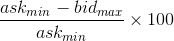# data.bitcoinity.org (beta version)

### Bitcoin exchangesName Rank Volume [BTC] Spread [%] Spread 10 BTC[%] Spread 100 BTC [%] Volatility (stddev) Trades per minute

#### Columns explanations

• Rank - check out the ranking system explanation, it is number estimating how big an exchange is, based on its order book
• Volume - sum of BTC traded in selected period
• Spread - bid/ask spread as percentage, calculated as• Spread 10 BTC - bid/ask spread with 10 BTC slippage, that is with 10 BTC worth of orders removed from bids and from asks
• Spread 100 BTC - bid/ask spread with 100 BTC slippage, that is with 100 BTC worth of orders removed from bids and from asks
• Volatility - price volatility calculated as standard deviation from all market trades. For longer periods it is average of hourly standard deviations (stddev calculated for each hour then averaged)
• Trades per minute - average number of trades per minute over the whole selected period